Work-Energy relation for a rigid body

Work of a force: One calculates the work of a force on a rigid body exactly the same as one would calculate the work of a force for a particle. For a rigid body, the velocity used is the velocity of the particle on the rigid body that the force is applied on.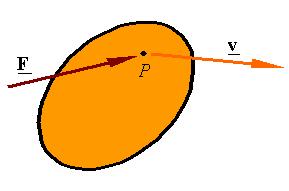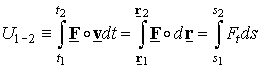Power of a couple: The power of a couple M applied on a rigid body having an angular velocity of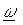is given by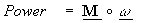Work of a couple: The work of a couple is the integral of its power over a time.

Work of a couple in 2-D: The work of a couple applied on a rigid body in two-dimensional motion is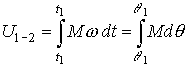Kinetic energy of a rigid body: The kinetic energy of a rigid body can be evaluated from one of the following equations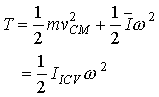Work-Energy relation: The work energy relation for a rigid body is given as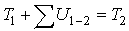As for a particle, one can use the potential energy to calculate the work of conservative forces and use the alternate form of the work-energy relation given by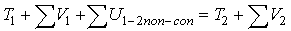Potential energy: The potential energy for a constant gravitational acceleration is given by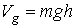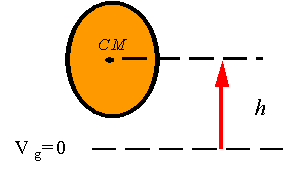where h is measured from a reference elevation to the center of mass of the rigid body.

The potential energy of a spring is calculated exactly the same as for a particle so that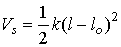where k is the spring constant, l is the current length, lo is the free length.Copy and distribute freely for personal use only

Department of Engineering Mechanics, University of Nebraska, Lincoln, NE 68588-0526